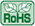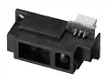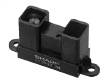# Products Lineup

## Distance Measuring Sensors <PSD type>Digital Output (Ta = 25°C)
Model No.
Detected
distance
(cm)
Features
Absolute
maximum ratings
Electro-optical characteristics*1
Vcc
(V)
Topr
(°C)
VOH
(V)
MIN.
VOL
(V)
MAX.
Dissipation current
Operating
(mA)
Standby
(µA)
13
Distance measuring sensor united with PSD*2,
infrared LED and signal processing circuit,
digital voltage output according to the measured distance
–0.3 to +7
–10 to +60
Vcc –0.3
0.6
MAX. 27
24
Distance measuring sensor united with PSD*2,
infrared LED and signal processing circuit,
digital voltage output according to the measured distance
–0.3 to +7
–10 to +60
Vcc –0.3
0.6
MAX. 40
80
Distance measuring sensor united with PSD*2,
infrared LED and signal processing circuit,
long distance measuring type digital voltage output according to the measured distance
–0.3 to +7
–10 to +60
Vcc –0.3
0.6
MAX. 50
*1 Vcc = 5 V
*2 PSD: Position Sensitive Detector
The model marked with ▲ may not be available in the near future. Contact with SHARP for details before use.

 Analog Output (Ta = 25°C)
Model No.
Distance
measuring
range
(cm)
Features
Absolute
maximum ratings
Electro-optical characteristics*1
Vcc
(V)
Topr
(°C)
VOH
(V)
MIN.
VOL
(V)
MAX.
Dissipation
current
Operating
(mA)
GP2Y0AF15 series
1.5 to 15
Distance measuring sensor united with PSD*2,
infrared LED and signal processing circuit,
short measuring cycle (16.5 ms), compact,
lineup of various connector shapes
–0.3 to +7
–10 to +60
VO (TYP.) = 0.4 V
(at L = 15 cm),
ΔVO (TYP.) = 2.3 V
(at L = 15 cm → 1.5 cm)
TYP. 17
2 to 15
Distance measuring sensor united with PSD*2,
infrared LED and signal processing circuit,
short measuring cycle (16.5 ms)
–0.3 to +7
–10 to +60
VO (TYP.) = 0.4 V
(at L = 15 cm),
ΔVO (TYP.) = 2.25 V
(at L = 15 cm → 2 cm)
TYP. 12
GP2Y0AF30 series
4 to 30
Distance measuring sensor united with PSD*2,
infrared LED and signal processing circuit,
short measuring cycle (16.5 ms), compact,
lineup of various connector shapes
–0.3 to +7
–10 to +60
VO (TYP.) = 0.4 V
(at L = 30 cm),
ΔVO (TYP.) = 2.3 V
(at L = 30 cm → 4 cm)
TYP. 17
4 to 30
Distance measuring sensor united with PSD*2,
infrared LED and signal processing circuit,
short measuring cycle (16.5 ms)
–0.3 to +7
–10 to +60
VO (TYP.) = 0.4 V
(at L = 30 cm),
ΔVO (TYP.) = 2.25 V
(at L = 30 cm → 4 cm)
MAX. 22
10 to 80
Distance measuring sensor united with PSD*2,
infrared LED and signal processing circuit
–0.3 to +7
–10 to +60
VO (TYP.) = 0.4 V
(at L = 80 cm),
ΔVO (TYP.) = 1.9 V
(at L: 80 cm → 10 cm)
MAX. 40
20 to 150
Distance measuring sensor united with PSD*2,
infrared LED and signal processing circuit,
long distance measuring type
–0.3 to +7
–10 to +60
VO (TYP.) = 0.4 V
(at L = 150 cm),
ΔVO (TYP.) = 2.05 V
(at L = 150 cm → 20 cm)
MAX. 50
100 to 550
Distance measuring sensor united with PSD*2,
infrared LED and signal processing circuit,
long distance measuring type
–0.3 to +7
–10 to +60
VO (TYP.) = 2.5 V
(at L = 100 cm),
ΔVO (TYP.) = 0.7 V
(at L = 100 cm → 200 cm)
TYP. 30
*1 Vcc = 5 V
*2 PSD: Position Sensitive Detector
The model marked with ▲ may not be available in the near future. Contact with SHARP for details before use.GP2Y0AF15 series GP2Y0AF30 series GP2Y0A21YK0F(GP2Y0D21YK0F,GP2Y0A41SK0FGP2Y0D413K0F▲: without mountinghole)GP2Y0A51SK0F GP2Y0D02YK0F(GP2Y0A02YK0F) GP2Y0A710K0F▲